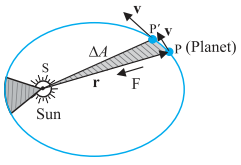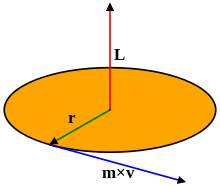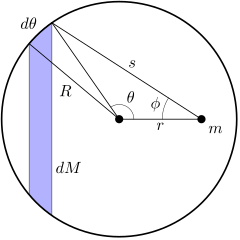# Gravitation - Online Test

Q1. According to Kepler’s Law of orbits:
Explaination / Solution:

The orbit of a planet around the Sun (or of a satellite around a planet) is not a perfect circle. It is an ellipse—a “flattened” circle. The Sun (or the centre of the planet) occupies one focus of the ellipse. A focus is one of the two internal points that help determine the shape of an ellipse. The distance from one focus to any point on the ellipse and then back to the second focus is always the same.

Q2. According to Kepler’s Law of areas,
Explaination / Solution:

Kepler law of areas describes the speed of a planet travelling in an elliptical orbit around the sun. A planet’s orbital speed changes, depending on how far it is from the Sun. The closer a planet is to the Sun, the stronger the Sun’s gravitational pull on it, and the faster the planet moves. The farther it is from the Sun, the weaker the Sun’s gravitational pull, and the slower it moves in its orbit. So, statement of Kepler's law of area is "The line joining the sun to the planet sweeps out equal areas in equal interval of time". i.e. areal velocity is constant.

Q3. According to Kepler’s Law of periods, The _______________ of the time period of revolution of a planet is proportional to the cube of the ___________ of the ellipse traced out by the planet
Explaination / Solution:

Kepler's 3rd Law: T= a3. Kepler's 3rd law is a mathematical formula. It means that if you know the period of a planet's orbit (T = how long it takes the planet to go around the Sun), then you can determine that planet's distance from the Sun (a is the length of the semimajor axis of the planet's orbit)

Q4.

The area A swept out by a planet of mass m in time interval t is related to the angular momentum by:

Explaination / Solution:The law of areas can be understood as the consequence of conservation of angular momentum which is valid for any central for A central force is such that the force on the planet is along the vector joining the sun and the planet. Let the sun be at the origin and let the position and momentum of the planet be denoted by r and p  respectively. Then the area swept out by the planet of mass m in the time interval Δt is given by ΔA = ½  (r × vΔt).

Hence ΔA/Δt    =½ (r × p)/m, (since  v=p/m)

=    L / (2 m)

where v is the velocity,  where v is the velocity,  L is the angular
momentum equal to   ( r  ×  p  ).  For a central force, which is directed along r, L is a constant

Q5.

Newton’s law of universal gravitation states that the gravitational force of attraction between any two particles of masses m1 and m2 separated by a distance r has the magnitude

Explaination / Solution:

According to Newton's law of universal gravitation, the force of attraction between two objects is directly proportional to the product of two masses and inversely proportional to the square of the distance between them

Q6. The direction of the universal gravitational force between particles of masses m1 and m2 is:
Explaination / Solution:

since gravitational force is attractive in nature, so if there are two particles of m& m2 .

So One Force will be on m1 which will be directed towards m 2. i.e

And other force wiil be on m2 which will be directed towards m 1 i.e

Clearly, It can be seen that  Because thsese forces are attracted to each other and direction of each force is opposite to other one.

Q7. A ‘central’ force is always directed
Explaination / Solution:The motion of a particle under a central force F always remains in the plane defined by its initial position and velocity.This may be seen by symmetry. Since the position r, velocity v and force F all lie in the same plane, there is never an acceleration perpendicular to that plane, because that would break the symmetry between "above" the plane and "below" the plane.

To demonstrate this mathematically, it suffices to show that the angular momentum of the particle is constant. This angular momentum L is defined by the equation

Q8.

The gravitational force on point mass m1 due to point mass m2, m3 & m4 is:

Explaination / Solution:

According to properties of gravitational force: force between the particles is independent of the presence or absence of other particles; so the principle of superposition is valid i.e. force on a particle due to a number of particles is the resultant of forces due to individual particles i.e.
Q9. To find the resultant gravitational force acting on the particle m due to a number of masses we need to use:
Explaination / Solution:

According to properties of gravitational force, gravitational force between the particles is independent of the presence or absence of other particles; so the principle of superposition is valid i.e. force on a particle due to number of particles is the resultant of forces due to individual particles i.e.
Q10. The force of attraction between a hollow spherical shell of uniform density and a point mass situated outside is just as if the entire mass of the shell is
Explaination / Solution:According to Shell's theorem, If a particle of mass m is located outside a spherical shell of mass M at, for instance, point P, the shell attracts the particle as though the mass of the shell were concentrated at its centre. Thus, as far as the gravitational force acting on a particle outside the shell is concerned, a spherical shell acts no differently from the solid spherical distributions of mass.